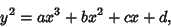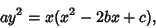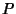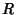## Cubic Curve

A cubic curve is an Algebraic Curve of degree 3. An algebraic curve over a Fieldis an equation, whereis a Polynomial inandwith Coefficients in, and the degree ofis the Maximum degree of each of its terms (Monomials).

Newtonshowed that all cubics can be generated by the projection of the five divergent cubic parabolas. Newton's classification of cubic curves appeared in the chapter Curves'' in Lexicon Technicum by John Harris published in London in 1710. Newton also classified all cubics into 72 types, missing six of them. In addition, he showed that any cubic can be obtained by a suitable projection of the Elliptic Curve(1)

where the projection is a Birational Transformation, and the general cubic can also be written as(2)

Newton's first class is equations of the form(3)

This is the hardest case and includes the Serpentine Curve as one of the subcases. The third class was(4)

which is called Newton's Diverging Parabolas. Newton's 66th curve was the Trident of Newton. Newton's classification of cubics was criticized by Eulerbecause it lacked generality. Plücker later gave a more detailed classification with 219 types.Pick a point, and draw the tangent to the curve at. Call the point where this tangent intersects the curve. Draw another tangent and call the point of intersection with the curve. Every curve of third degree has the property that, with the areas in the above labeled figure,(5)

(Honsberger 1991).

References

Honsberger, R. More Mathematical Morsels. Washington, DC: Math. Assoc. Amer., pp. 114-118, 1991.

Newton, I. Mathematical Works, Vol. 2. New York: Johnson Reprint Corp., pp. 135-161, 1967.

Wall, C. T. C. Affine Cubic Functions III.'' Math. Proc. Cambridge Phil. Soc. 87, 1-14, 1980.

Westfall, R. S. Never at Rest: A Biography of Isaac Newton. New York: Cambridge University Press, 1988.

Yates, R. C. Cubic Parabola.'' A Handbook on Curves and Their Properties. Ann Arbor, MI: J. W. Edwards, pp. 56-59, 1952.